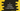# 3 different C program to check for spy number## C program to check if a number is a spy number or not:

In this C programming tutorial, we will learn how to check if a number is a spy number or not. We will learn different ways to check for a spy number in this tutorial. The program will ask the user to enter a number. It will check if the number is a spy number or not and it will print one result.

### What is a spy number:

If the sum of digits of a number is equal to the product of all digits of that number, it is called a spy number.

For example, 123 is a spy number because the sum of its digits is 1+2+3 = 6 and the product of its digits is 123 = 6. Both are equal.

Similarly, 1234 is not a spy number the sum of its digits is 1+2+3+4 = 10 and the product of its digits is 1234 = 24*.

### Algorithm to check if a number is a spy number:

We can use the below algorithm to check if a number is spy or not:

1. Take the number as input from the user.
2. Initialize two variables as 0 to store the sum and product of digits of the number.
3. Run one loop. It will run till the number is greater than 0.
1. On each iteration of the loop, get the rightmost digit of the number. We can use modulo, % to get the last digit.
2. Add the digit to the current sum variable and multiply the digit to the current product variable.
3. Remove the last digit of the number.
4. The loop will stop once all digits are removed, i.e. it will become 0.
5. Compare the values of sum and product. If both are equal, this is a spy number. Else, it is not.

### Method 1: C program to check if a number is a spy number or not by using a for loop:

This program will use a for loop to check if a number is a spy number or not.

``````#include <stdio.h>

int main()
{
int no;
int product = 1, sum = 0, lastDigit;

printf("Enter a number: ");
scanf("%d", &no);

for (; no > 0;)
{
lastDigit = no % 10;

product *= lastDigit;
sum += lastDigit;

no /= 10;
}

if (product == sum)
{
printf("It is a spy number\n");
}
else
{
printf("It is not a spy number\n");
}
}``````
• The integer variable no is used to hold the user input number. We have created three more integer variables product, sum and lastDigit to hold the final product of all digits of the number, sum of all digits of the number and last digit of the number.
• It asks the user to enter a number. It reads and store that value in the no variable.
• The for loop will run until the value of no is positive.
• On each iteration of the loop:
• It finds the last digit of the number and assign it to lastDigit.
• The last digit is multiplied to the product variable and it is added to the sum variable.
• At the end of each iteration of the loop, it removes the last digit from the number by dividing the number by 10.
• Once the loop ends, it compares the product and sum values. If both are equal, it is a spy number. Else, it is not a spy number.

If you run this program, it will print output as like below:

``````Enter a number: 123
It is a spy number

Enter a number: 234
It is not a spy number``````

### Method 2: C program to check if a number is spy number or not by using a while loop:

We can use a while loop to check if a number is spy or not. It will work in a similar way. Let’s re-write the above program by using while loop:

``````#include <stdio.h>

int main()
{
int no;
int product = 1, sum = 0, lastDigit;

printf("Enter a number: ");
scanf("%d", &no);

while (no > 0)
{
lastDigit = no % 10;

product *= lastDigit;
sum += lastDigit;

no /= 10;
}

if (product == sum)
{
printf("It is a spy number\n");
}
else
{
printf("It is not a spy number\n");
}
}``````

It will print similar results.

You can see that only the loop is changed in this program.

### Method 3: C program to check if a number is spy number or not by using a separate function:

Let’s use a separate function to check if a number is spy number or not. This function will take the number as input from the user and it will return one value based on it is a spy number or not.

``````#include <stdio.h>

int isSpy(int no)
{
int product = 1, sum = 0, lastDigit;
while (no > 0)
{
lastDigit = no % 10;

product *= lastDigit;
sum += lastDigit;

no /= 10;
}

return product == sum;
}

int main()
{
int no;

printf("Enter a number: ");
scanf("%d", &no);

if (isSpy(no))
{
printf("It is a spy number\n");
}
else
{
printf("It is not a spy number\n");
}
}``````
• isSpy is the method used to check if a number is a spy number or not. It takes the number as its parameter and returns 1 if it is a spy number or 0 if it is not.
• It is using the same algorithm we used in the previous examples.
• isSpy is called from the main method and based on its return value, one message is printed on the console.

It will print similar output. The advantage of using a separate method is that we can call this method from different parts of a program without repeating the code.